#### Previous topic

XSect Documentation

#### Next topic

xsect.calc.CrossSection

# Cross Section Calculations (xsect.calc)¶

Contains functions and classes for calculating and storing cross sectional properties for structural members.

## Cross Section Classes¶

The below class provides a container for storing cross sectional properties.

 CrossSection(name, area[, width, height, …]) A class representing a member cross section.

## Boundary Functions¶

The following functions may be used to calculate cross sectional properties based on sections defined by boundary points.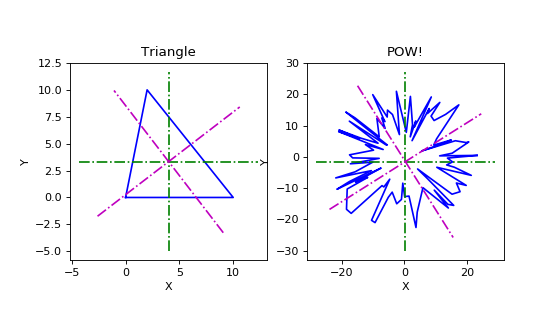rotate2(x, angle[, origin]) Rotates an array of 2D row vectors by an angle about the specified origin. close_points(points) If the input points do not form a closed polygon, closes the polygon and returns the result. area(points) Returns the area enclosed by the input points. centroid(points) Returns the (x, y) centroid coordinates for the shape defined by the input boundary points. inertias(points[, origin]) Returns the area moment of inertias for the shape defined by the input boundary points. principal_inertias(points) Returns the area moment of inertias about the principal axes. principal_angles(points) Returns the principal angles. gyradii(points) Returns the radii of gyration about the x and y axes. principal_gyradii(points) Returns the radii of gyration about the principal axes. extreme_fibers(points) Returns the extreme fibers from the x and y axes. principal_extreme_fibers(points) The returns the extreme fibers from the principal axes. elast_sect_mod(points) Returns the section modulii about the x and y axes. principal_elast_sect_mod(points) Returns the section modulii about the principal axes. plot_section(points[, ax, title, symbols]) Plots the cross section defined by the input boundary points. section_summary(points) Returns a dictionary with a summary of cross sectional properties for the shape defined by the input boundary points.

## Multi-Boundary Functions¶

The following functions may be used to calculate cross sectional properties for composite sections composed of multiple elements which are defined by boundary points.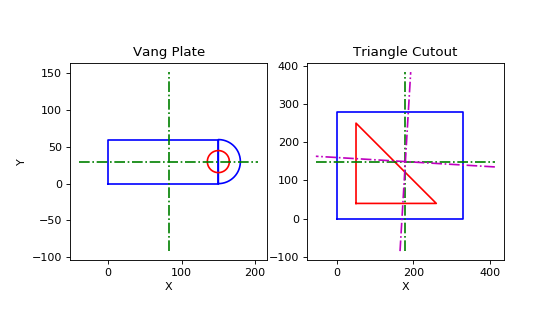multi_area(add[, subtract]) Returns the total cross sectional area for multiple shapes. multi_centroid(add[, subtract]) Returns the centroid for cross sections including multiple shapes. multi_inertias(add[, subtract, origin]) Calculates the area moment of inertias for cross sections including multiple shapes. multi_principal_inertias(add[, subtract]) Calculates the principal area moment of inertias for multiple shapes. multi_principal_angles(add[, subtract]) Calculates the angles from the x-axis to the principal axes. multi_gyradii(add[, subtract]) Calculates the radii of gyrations about the x and y axes. multi_principal_gyradii(add[, subtract]) Calculates the radii of gyrations about the principal axes. multi_extreme_fibers(add[, subtract]) Calculates the extreme fibers from the x and y axes. multi_principal_extreme_fibers(add[, subtract]) Calculates the extreme fibers from the principal axes. multi_elast_sect_mod(add[, subtract]) Calculates the section modulii about the x and y axes. multi_principal_elast_sect_mod(add[, subtract]) Calculates the section modulii about the principal axes. multi_plot_section(add[, subtract, ax, …]) Plots a cross section consisting of multiple shapes. multi_section_summary(add[, subtract]) Returns a dictionary with a summary of cross sectional properties for the composite shape.

## Round Functions¶

The following functions may be used to calculate cross sectional properties for round sections, such as rounds and pipes.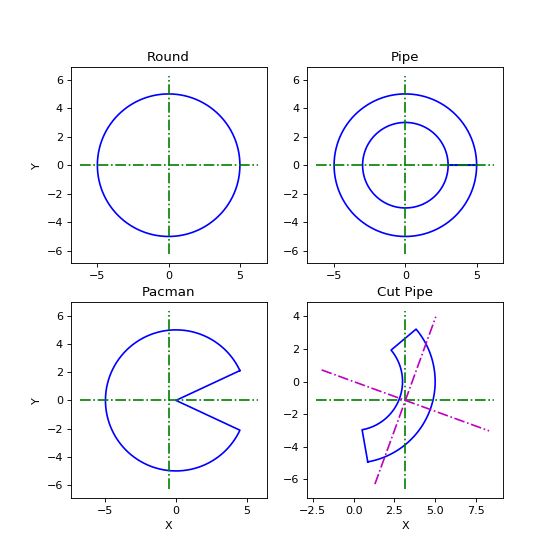round_area(diameter[, thickness]) Returns the cross sectional area for a round or pipe. round_inertia(diameter[, thickness]) Returns the area inertia for a round or pipe. round_gyradius(diameter[, thickness]) Returns the radius of gyration for a round or pipe. round_sect_mod(diameter[, thickness]) Returns the section modulus for a round or pipe. round_points(diameter[, thickness, start, …]) Returns an array of boundary points for a round or pipe. round_summary(diameter[, thickness, start, …]) Returns a dictionary with a summary of cross sectional properties for the round.

## Polygon Functions¶

The following functions may be used to calculate the cross sectional properties for solid or thin walled polygon sections.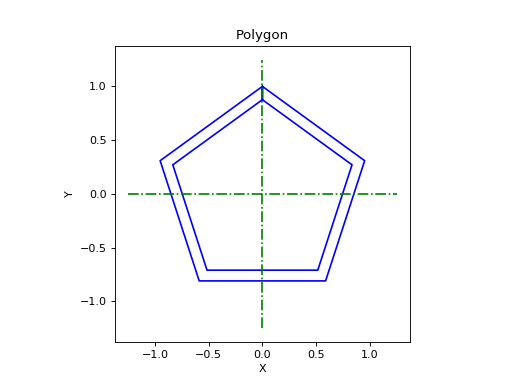polygon_points(n, radius[, thickness, …]) Returns an array of boundary points for a polygon. polygon_summary(n, radius[, thickness, …]) Returns a dictionary with a summary of polygon section properties.

## Angle Functions¶

The following functions may be used to calculate cross sectional properties for angle or “L” shape sections.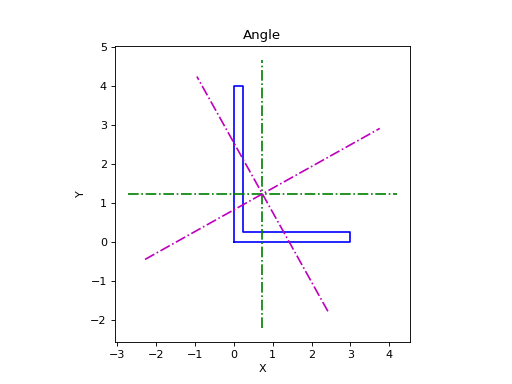angle_points(leg1, leg2, thickness1[, …]) Returns an array of angle boundary points of shape (N, 2). angle_summary(leg1, leg2, thickness1[, …]) Returns a dictionary with a summary of angle section properties.

## Double Angle Functions¶

The following functions may be used to calculate cross sectional properties for double angle or “2L” shape sections.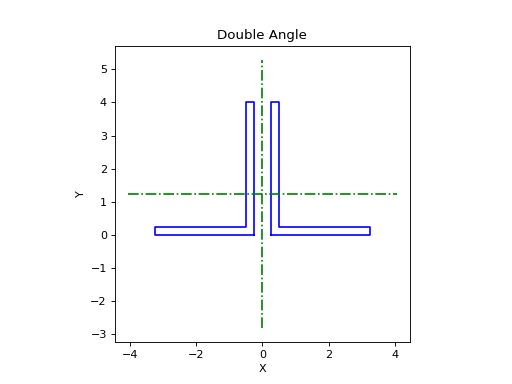double_angle_points(leg1, leg2, thickness1) Returns an array of double angle boundary points of shape (N, 2). double_angle_summary(leg1, leg2, thickness1) Returns a dictionary with a summary of double angle properties.

## Cruciform Functions¶

The following functions may be used to calculate cross sectional properties for cruciform or “4L” shape sections.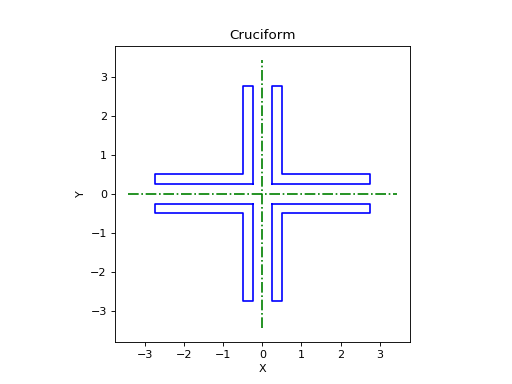cruciform_points(leg1, leg2, thickness1[, …]) Returns an array of cruciform boundary points of shape (N, 2). cruciform_summary(leg1, leg2, thickness1[, …]) Returns a dictionary with a summary of cruciform properties.

## I-Beam Functions¶

The following functions may be used to calculate cross sectional properties for I-beam sections.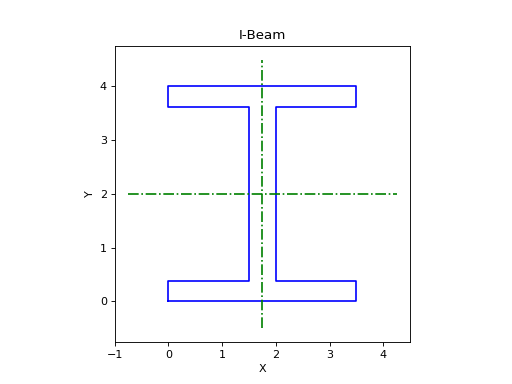i_beam_points(height, width, …) Returns an array of I-beam boundary points of shape (N, 2). i_beam_summary(height, width, …) Returns a dictionary with a summary of I-beam section properties.

## T-Beam Functions¶

The following functions may be used to calculate cross sectional properties for T-beam sections.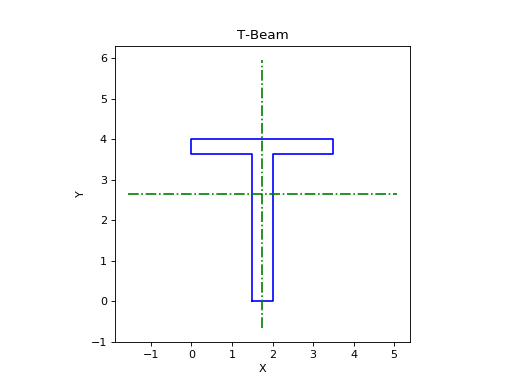t_beam_points(height, width, …) Returns an array of T-beam boundary points of shape (N, 2). t_beam_summary(height, width, …) Returns a dictionary with a summary of T-beam section properties.